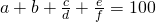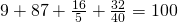Pandigital fraction sums

April 14th, 2009 | Categories: general math | Tags:

Over at Wild about Math, Sol introduced pandigital fraction sums of the formI call these sums pandigital because the left hand side contains every digit from 0-9 once and once only.  Sol challenged his readers to come up with more equations of this type and so I had a crack using Mathematica.

In order to keep the number of solutions down (and to keep them as close as possible to Sol’s original examples), I only considered sums with four terms – 2 of which were integers and 2 of which were fractions. In other words sums of the formMy next simplification was to only consider numerators and denominators of up to 2 digits each so fractions such as 123/456 were immediately out.  As you might expect, I also didn’t count trivial re-arrangements of the terms as separate solutions.

Finally, I didn’t insist that the fractions were written in their lowest terms so, for example, 38/76 is allowed even though it could be rewritten as 1/2.  Since one of Sol’s original examples contained such a fraction, I didn’t want to remove them.  This also meant that I allowed fractions that could be rewritten as integers such as 48/16.

Even with all of these restrictions in place I came up with 580 solutions!  One of which isInterestingly, (unless I have made a mistake) it seems that if you insist on having fractions in their lowest terms then there are no solutions at all!

Does anyone out there have anything to add to this little problem?  Have I messed up and missed a solution or is one of my solutions incorrect?  Have I missed something obvious?  Are there more interesting variations?  Anything?

1. Here’s a proof that there are no solutions with both fractions in lowest terms. Say there is such a solution. The two fractions must add up to an integer. But two fractions which add up to an integer, when written in lowest terms, will have the same denominator. (Two fractions a/b and (b-a)/b which add up to 1 will have the same denominator, since gcd(b,a) = gcd(b, b-a).) Since there can be no repeated digits, the two denominators can’t be the same.

Now, it’s possible to have a sum of three fractions in lowest terms which is an integer, with all three denominators distinct. For example, 1/2 + 4/3 + 7/6 is an integer, namely 3. So there may be solutions to an analogous problem with three fractions, where all three are in lowest terms.

2. hey Mike,

Rob and I were working on this exact problem as well. We explored all possible closed forms, and Rob thinks he has the correct answer. We’ll probably post it sometime soon. Needless to say, I don’t think his answer matched yours . . .

Quan

3. Hi Quan

Well I hope that my solutions appear as a subset of yours at least :)
Looking forward to seeing the post at Blinkdagger.

Cheers,
Mike

4. Thanks for the proof Michael – maybe Quan and Rob will come up with some solutions with 3 fractions in their lowest terms…